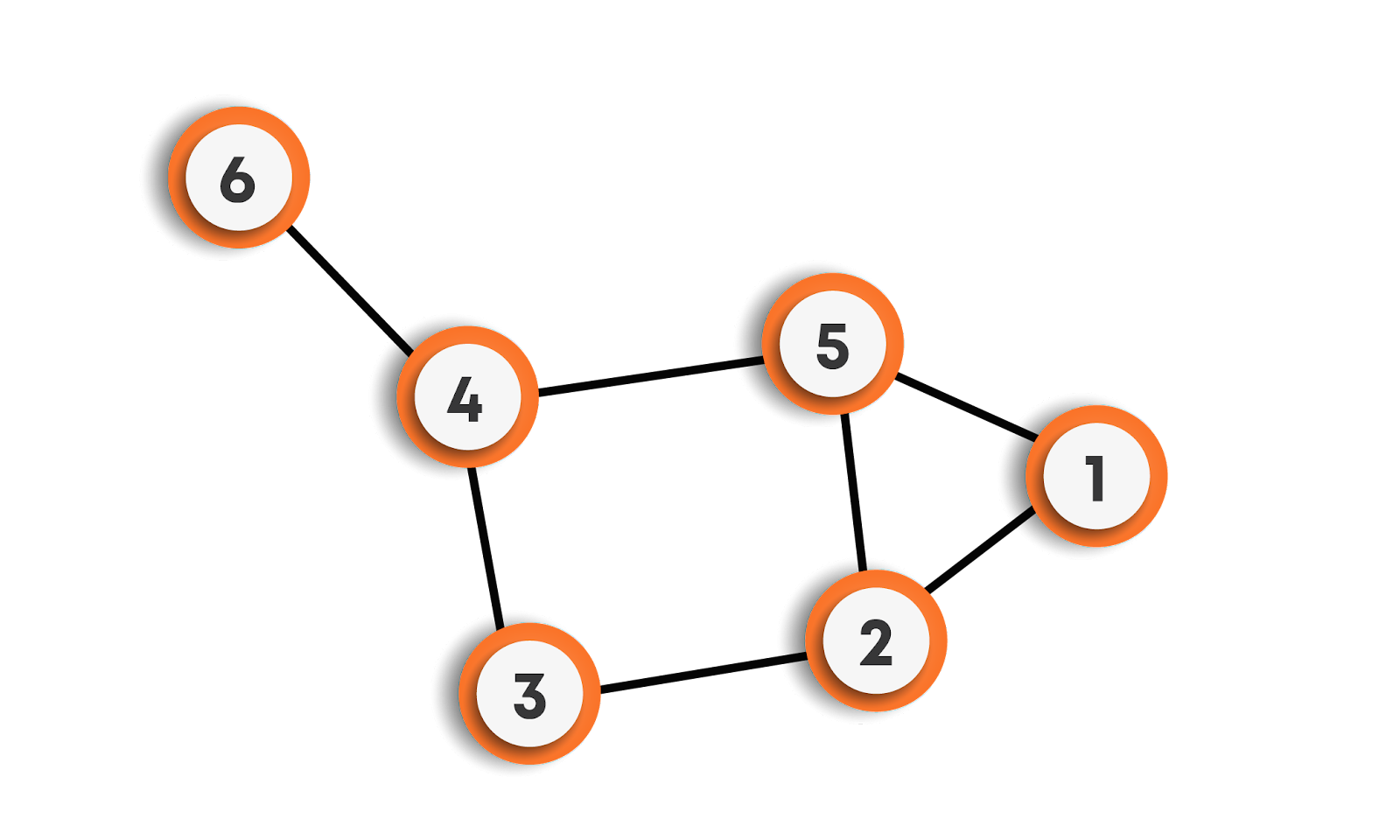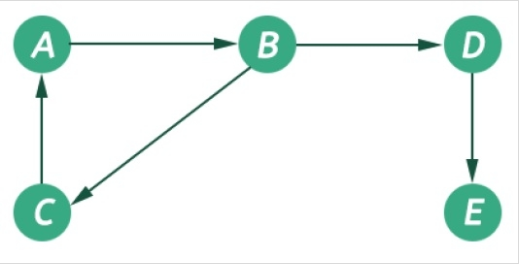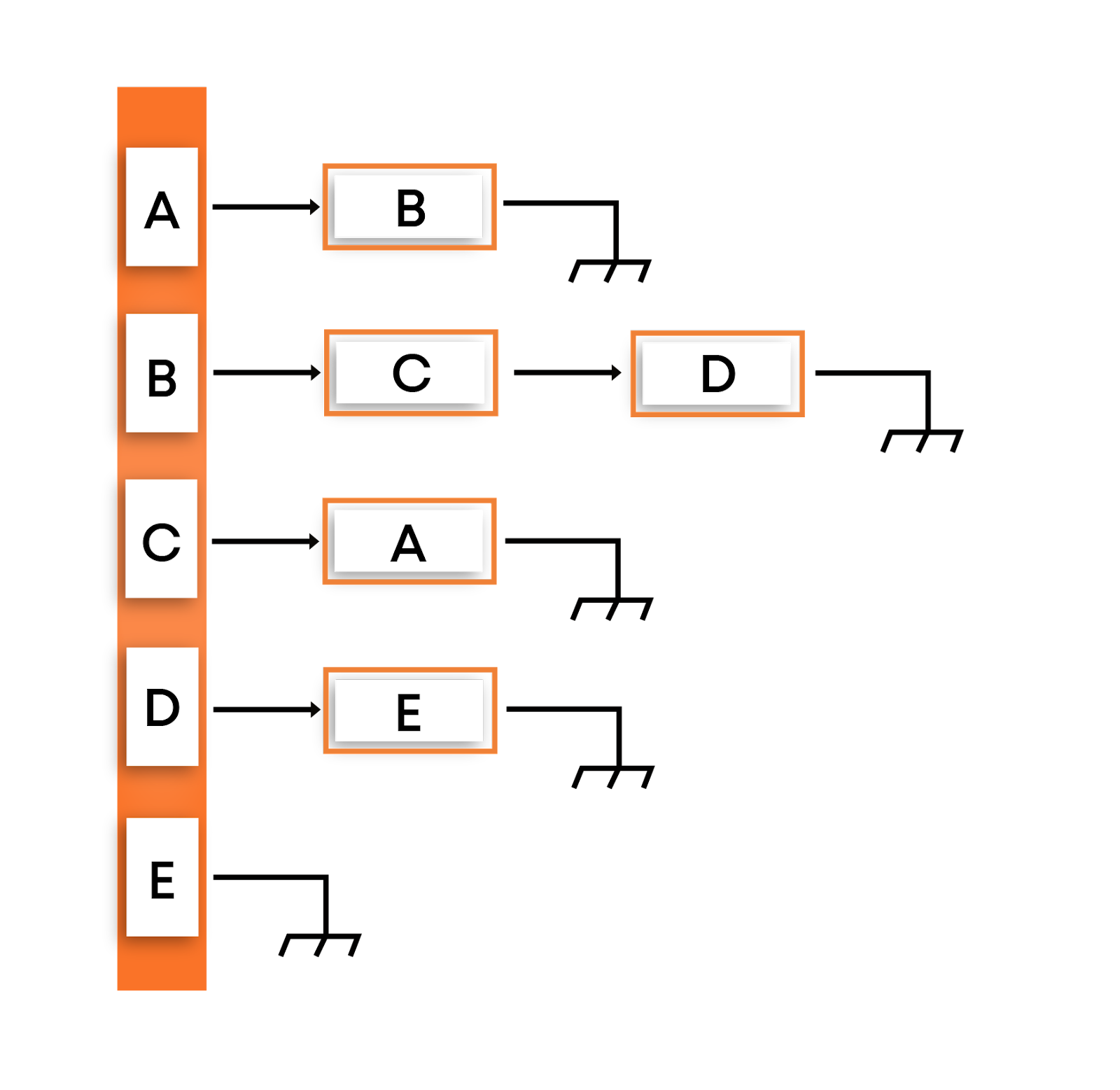# Implementation of Graph in Python

## Introduction

Everyone works hard to crack their dream company, prepare Data Structures and Algorithms, Computer Science Fundamentals, and solve interview problems.(Source: giphy)

One important topic one should be well versed in is Graphs.

Questions related to Graphs are asked in all the major product-based companies. Also, Graphs are used everywhere, and directed graphs are used in Google's page ranking algorithms. Social networking sites like Facebook, Linkedin use graphs to represent different users as vertices and edges to represent the connections between them.  Therefore it's quite important to have a solid understanding of Graphs.

The first step to study Graphs is to know the basic terminologies related to graphs. You may refer to this article for more information. After knowing the basic terminologies, it’s time to implement your Graph. This article will discuss the implementation of graphs in Python. Before moving on to the implementation, it’s necessary to quickly understand Graphs.

## A Brief Introduction to Graphs

A graph is a non-linear data structure that consists of nodes and edges. Formally, a graph can be defined as an ordered set G(V, E) where V represents the vertices and E represents the set of edges that are used to connect these vertices.In the above graph, the set of nodes are {1, 2, 3, 4, 5, 6} and the set of edges are {1-2, 1-5, 5-2, 2-3, 3-4, 4-5, 4-6}.

Graphs may be directed or undirected graphs. A directed graph is a set of vertices/nodes connected by edges, with each node having a direction associated with it. Edges are represented by arrows pointing to the direction in which the graph can be traversed. If there is an edge between vertex 0 and 1 with an arrow pointing towards 1, then the graph can be traversed from 0 to 1 and not from 1 to 0. In contrast, an undirected graph can be traversed in either direction. The edges are bidirectional. If there is an edge between vertex 0 and 1, then the graph can be traversed from 0 to 1 and from 1 to 0.

Some graphs have weights associated with edges between two vertices. These graphs are called Weighted Graphs.

Implementation of Graph can be done by using either an adjacency list or an adjacency matrix. Each of the two representations has its pros and cons; the choice of a particular graph representation depends on the requirements.

## Implementation of Graph in Python - Using Adjacency Matrix

A graph can be represented using an adjacency Matrix. An adjacency matrix is a square matrix of size V * V, where V is the number of vertices in the graph. The values in the matrix show whether a given pair of nodes are adjacent to each other in the graph structure or not. In the adjacency matrix, 1 represents an edge from node X to node Y, while 0 represents no edge from X to Y.The adjacency matrix representation of the above-directed graph is:Note that for an undirected graph, the adjacency matrix is always symmetric. For a weighted graph, instead of 0 and 1, the value of weight w is used to indicate that there is an edge from i to j.

### Implementation

The Implementation of Graphs in Python using Adjacency Matrix is done in the following program:

# Adjacency Matrix representation of a graph

class Graph:

# self represents the instance of the class.

# By using the “self” keyword we can access the

# attributes and methods of the class in python.

# It binds the attributes with the given arguments.

# This constructor is used to initialize the adjacecny matrix

# with 0.

def __init__(self, vertices):

self.V = vertices

self.graph = [[0 for column in range(vertices)]

for row in range(vertices)]

# This function prints the adjacency matrix of the graph

# Due to two nested loops, it is O(V^2)

def printGraph(self):

for i in range(self.V):

for j in range(self.V):

print(self.graph[i][j], end = " ")

print()

# This function is used to add an edge

# between vertices v and w.

# This implementation is for undirected graph

print("Adding an edge between", v , "and", w , "and between", w , "and", v)

self.graph[v][w] = 1

self.graph[w][v] = 1

# This function is used to add a

# vertex to the graph.

self.V += 1

for i in range(self.V):

self.graph[i].append(0)

self.graph.append([0 for column in range(self.V)])

if __name__ == "__main__":

# Initialize the graph with 5 vertices

g = Graph(5)

# An edge between 0 and 1 and between 1 and 0 will be created

# An edge between 0 and 2 and between 2 and 0 will be created

# An edge between 1 and 2 and between 2 and 1 will be created

# An edge between 2 and 0 and between 2 and 0 will be created

# An edge between 2 and 3 and between 3 and 2 will be created

# An edge between 3 and 4 and between 4 and 3 will be created

g.printGraph()

The output of the above program is:

The time complexity of the addEdge() function is O(1) and the space complexity is O(V^2).

• Insertion and deletion of an edge can be done in O(1) time.
• We can easily determine if two edges are adjacent to each other in constant time complexity.

• The memory consumption of adjacency matrix representation is of the order of O(V^2), where V is the number of vertices in the graph, which is way too high as compared to Adjacency Lists
• Traversal of a graph requires O(V^2) time complexity.

## Implementation of Graph in Python- Using Adjacency List

A graph can also be represented in the form of an adjacency list. An adjacency list represents a graph as an array of linked lists, wherein the index of the array represents a vertex, and each element of the linked list represents other vertices that form an edge with the vertex.The adjacency list representation of the above graph is:In the adjacency list representation shown above, the vertices A, B, C, D, and E will be stored in a list, and each vertex will have a separate list containing the vertices that form an edge with the vertex.

### Implementation

The below program shows the implementation of Graphs in Python using adjacency list representation.

# A utility function to add a vertex to the graph

'''

The purpose of using global variables inside all the

functions defined below is that global variables can be

used both inside and outside the functions

'''

global graph

# This variable is used to count the number of vertices in the graph

global vertexCount

# If the vertex already exists in the graph

if vertex in graph:

print("Vertex ", vertex, " already exists in the graph ")

# Otherwise create a new list for this vertex and increase the count of

# vertices in the vertexCount variable

else:

vertexCount += 1

graph[vertex] = []

# A utility function to add an edge between

# vertex source and destination

global graph

# Check if the source vertex exists in the graph

if source not in the graph:

print("Source vertex ", source, " does not exist in the graph")

# Check if the destination vertex exists in the graph

elif destination not in graph:

print("Destination vertex ", destination,

" do not exist in the graph")

# If both the source and destination vertex exists in the

# graph, an edge can be added.

else:

# Create a new list by passing in the data of the destination vertex

temp = [destination]

# The append() method adds a single item to the list. A new list is

# not created. Instead, the original list is modified by adding the new

# item to the rear of the original list

graph[source].append(temp)

# A utility function to print the adjacency list representation of

# a graph

def printGraph():

global graph

# Pick each vertex

for vertex in graph:

# Pick all the vertices that form an edge with the picked vertex above.

for edges in graph[vertex]:

print(vertex, "->", edges)

if __name__ == '__main__':

# Initializing the graph with an empty list

# This will store all the vertices

graph = {}

# This variable is used to store the count of vertices in the graph

vertexCount = 0

# Creating 5 vertices in the graph. Initially none

# of them is connected to each other or have an edge

# between them

# Adding the edges between source and destination vertices

'''

1 --------> 2

'''

'''

1 ---------> 2

|

|

\|/

3

'''

'''

1 ----------> 2

|

|

\|/

4 <---------- 3

'''

'''

1 ----------> 2

/|\            |

|             |

|            \|/

4 <---------- 3

'''

# Function call to printGraph() function

printGraph()

print("Internal representation of graph is: ", graph)

The output of the above program is:

The time complexity of the addVertex() is O(1), addEdge() is O(1) as append() operation takes constant time. The printGraph() function however takes O(V*E) time.

The space complexity of the above program is O(V + E), where V is the number of vertices and E is the number of edges. The space complexity of the above program will be O(V^2) in the case of a complete graph.

• The representation is quite easy to follow and implement. It clearly shows the adjacent nodes of a particular node.
• For graphs with a small-to-moderate number of edges, the Adjacency list consumes less memory of the order of [O(V + E), where V is the number of vertices and E is the number of edges] as compared to the Adjacency matrix. Therefore it is a preferred choice for a graph with a small-to-moderate number of nodes.

• It takes linear time to determine whether there is an edge from vertex ‘i’ to ‘j’ as compared to the constant time in the case of the adjacency matrix.
• Also, in the case of graphs with a high number of vertices and edges, the memory consumption O(V^2) is comparable to the Adjacency Matrix. So the adjacency matrix is a preferred choice in such cases.

Q1) How is the graph implemented?

Ans 1) A graph can be implemented by using an edge list.

• Edge List: An edge list is a list or array of all the edges in a graph. To represent an edge, we just have an array of two vertex numbers or an array of objects containing the vertex number of the vertices that the edges are incident on.
• Adjacency Matrix: An adjacency matrix is a matrix of size V*V where V is the number of vertices in the graph. The entry in row i and column j is 1 if there is an edge from i to j; otherwise, it's 0.
• Adjacency List: It’s a combination of an Edge list and an adjacency matrix. For a vertex,v, store the vertices that are adjacent to it.

Q2) How is a graph traversed?

Ans 2) Traversal of a graph means visiting all the vertices and edges of the graph. Graph traversal can be done in one of the following ways.

2. Depth First Search.

## Conclusion

Graphs are one of the most important data structures from an interview perspective in product-based companies. This article discusses the implementation of Graph in Java. With this done, you must practice more and more questions related to Graphs on Codestudio.

You must check out Codestudio to upskill yourself. Codestudio is an excellent platform for curated guided paths, to practice interview problems, and to read blogs curated by experts. Remember Practice is the Key !!